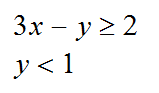5262 Graphing Two Linear Inequalities

6 Questions | Total Attempts: 104SettingsFinding the points of intersection for two inequalities is important for accurate graphing. Simultaneous equations will give you the point of intersection of two inequalities. Use the coordinate convention to name the points of intersection. (1, 3). . . Note. . . Comma space.

• 1.
What is the point of intersection of the two linear inequalities above?Give you answer in coordinate form (3, 2)
• 2.
What is the point of intersection of the two linear inequalities above?Give you answer in coordinate form (3, 2)
• 3.
What is the point of intersection of the two linear inequalities above?Give you answer in coordinate form (3, 2)
• 4.
What is the point of intersection of the two linear inequalities above?Give you answer in coordinate form (3, 2)
• 5.
What is the point of intersection of the two linear inequalities above?Give you answer in coordinate form (3, 2)
• 6.
What is the point of intersection of the two linear inequalities above?Give you answer in coordinate form (3, 2)
• 7.
What is the point of intersection of the two linear inequalities above?Give you answer in coordinate form (3, 2)
• 8.
What is the point of intersection of the two linear inequalities above?Give you answer in coordinate form (3, 2)
• 9.
What is the point of intersection of the two linear inequalities above?Give you answer in coordinate form (3, 2)
• 10.
What is the point of intersection of the two linear inequalities above?Give you answer in coordinate form (3, 2)
• 11.
What is the point of intersection of the two linear inequalities above?Give your answer in coordinate form (3, 2)
• 12.
What is the point of intersection of the two linear inequalities above?Give you answer in coordinate form (3, 2)
• 13.
What is the point of intersection of the two linear inequalities above?Give you answer in coordinate form (3, 2)
• 14.
What is the point of intersection of the two linear inequalities above?Give you answer in coordinate form (3, 2)
• 15.
What is the point of intersection of the two linear inequalities above?Give you answer in coordinate form (3, 2)
• 16.
What is the point of intersection of the two linear inequalities above?Give you answer in coordinate form (3, 2)
• 17.
What is the point of intersection of the two linear inequalities above?Give you answer in coordinate form (3, 2)
• 18.
What is the point of intersection of the two linear inequalities above?Give you answer in coordinate form (3, 2)
• 19.
What is the point of intersection of the two linear inequalities above?Give your answer in coordinate form (3, 2)
• 20.
What is the point of intersection of the two linear inequalities above?Give you answer in coordinate form (3, 2)Back to top